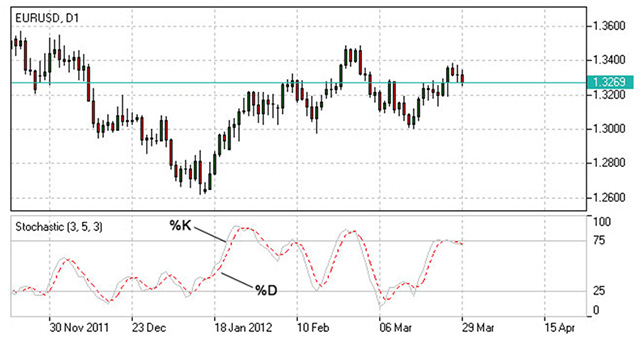Stochastic Oscillator | Stochastic Indicator | Stochastic Formula | How to Use Stochastic Oscillator | IFCM UK

# Stochastic Oscillator - What is Stochastic

Stochastic indicator is introduced by George Lane to identify price trend direction and possible reversal points by determining the place of the current close price in the most recent price range, as in a sustainable uptrend close prices tend to the higher end of the range and to the lower end in a downtrend.

## How to use in trading platform

Confirm the theory on practice
Once opened Demo you will be supplied with educational materials and online support

## How to Use Stochastic Oscillator

The Stochastic oscillator allows to identify possible overbought and oversold areas, but should be considered within trend analysis:

• Generally if the Stochastic Oscillator climbs above 75, the asset may be overbought;
• If the Stochastic Oscillator drops below 25, the asset may be oversold.

Leaving extreme areas the indicator may suggest possible turning points:

• Crossing the overbought boundary from above, the Stochastic signals a possible sell opportunity;
• Crossing the oversold boundary from below, the Stochastic signals a possible buy opportunity.

Crossovers of the indicator with its smoothened signal line, usually a 3-period moving average, may also detect deal opportunities:

• The Stochastic Oscillator suggests going long when crossing the signal line from below;
• The Stochastic Oscillator suggests going short when crossing the signal line from above.

Convergence/divergence patterns may indicate possible trend weakness:

• If the price climbs to a new high, but the indicator does not, that may be a sign of the uptrend weakness;
• If the price falls to a new low, but the indicator does not, that may be a sign of the downtrend weakness.Stochastic Indicator

Stochastic system is based on the observation that in an uptrend closing prices tend to be near the upper end of the price range, and in a downtrend the closing prices tend to be near the lower end of the price range.

In the Stochastic strategy two lines - the %K line and the %D line – are used. The K line is faster and the D line is slower. These lines oscillate from 0 to 100 on the vertical scale. The major signal to consider is the divergence between the D line and the price of the underlying market. When the D line is over 80 and forms two declining peaks with prices moving higher, a bearish divergence occurs. When the D line is below 20 and forms two rising bottoms with prices moving lower, a bullish divergence takes place. Thus, the actual buy and sell signals are triggered when the K line crosses the D line. A sell signal is generated when the K line crosses below the D line from above the 80 level. Accordingly, a buy signal is generated when the K line crosses above the D line bellow the 20 level.

## Stochastic Oscillator Formula (Calculation)

The stochastic oscillator is calculated by dividing the difference between the last closing price and the low price over n periods into the difference between the high and low prices over n periods. To get an absolute range from 100 to 0, the fraction should be multiplied by 100. The number of time periods of the oscillator is set by a trader based on his trading strategy.

```Stochastic = 100 x ((C – L)/(H – L));
Signal = average of the last three Stochastic values;

where:
C – latest close price;
L – the lowest price over a given period;
H – the highest price over a given period.```